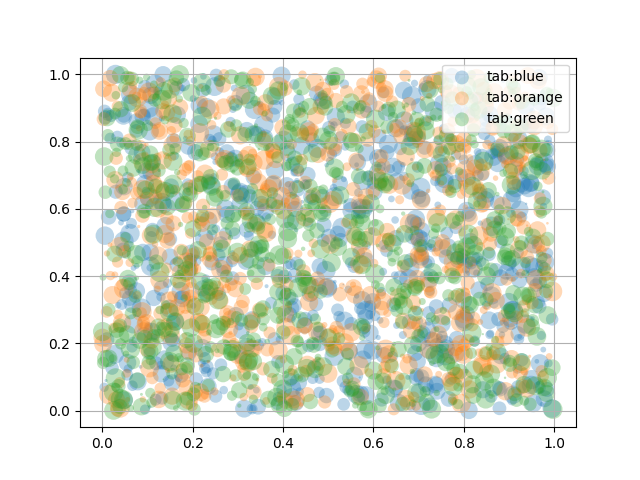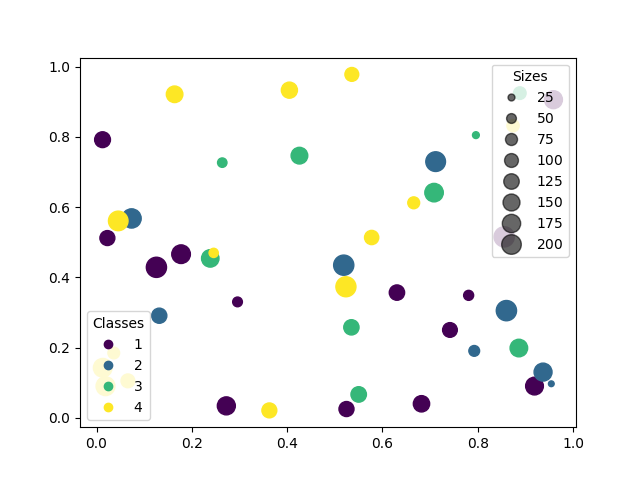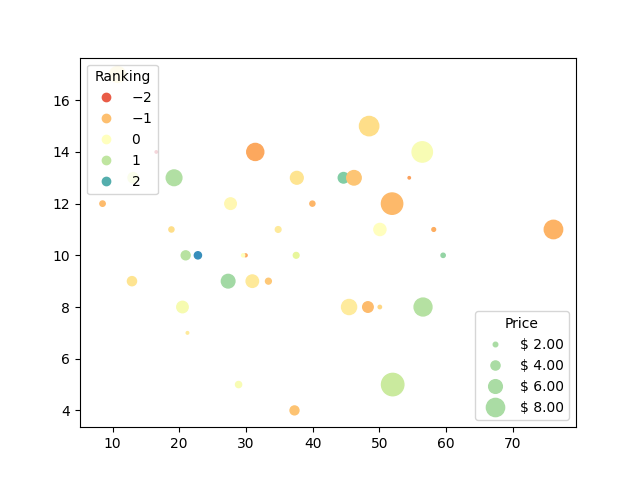# Scatter plots with a legend#

To create a scatter plot with a legend one may use a loop and create one scatter plot per item to appear in the legend and set the label accordingly.

The following also demonstrates how transparency of the markers can be adjusted by giving alpha a value between 0 and 1.

import numpy as np
import matplotlib.pyplot as plt

np.random.seed(19680801)

fig, ax = plt.subplots()
for color in ['tab:blue', 'tab:orange', 'tab:green']:
n = 750
x, y = np.random.rand(2, n)
scale = 200.0 * np.random.rand(n)
ax.scatter(x, y, c=color, s=scale, label=color,
alpha=0.3, edgecolors='none')

ax.legend()
ax.grid(True)

plt.show()## Automated legend creation#

Another option for creating a legend for a scatter is to use the PathCollection.legend_elements method. It will automatically try to determine a useful number of legend entries to be shown and return a tuple of handles and labels. Those can be passed to the call to legend.

N = 45
x, y = np.random.rand(2, N)
c = np.random.randint(1, 5, size=N)
s = np.random.randint(10, 220, size=N)

fig, ax = plt.subplots()

scatter = ax.scatter(x, y, c=c, s=s)

# produce a legend with the unique colors from the scatter
legend1 = ax.legend(*scatter.legend_elements(),
loc="lower left", title="Classes")

# produce a legend with a cross-section of sizes from the scatter
handles, labels = scatter.legend_elements(prop="sizes", alpha=0.6)
legend2 = ax.legend(handles, labels, loc="upper right", title="Sizes")

plt.show()Further arguments to the PathCollection.legend_elements method can be used to steer how many legend entries are to be created and how they should be labeled. The following shows how to use some of them.

volume = np.random.rayleigh(27, size=40)
amount = np.random.poisson(10, size=40)
ranking = np.random.normal(size=40)
price = np.random.uniform(1, 10, size=40)

fig, ax = plt.subplots()

# Because the price is much too small when being provided as size for s,
# we normalize it to some useful point sizes, s=0.3*(price*3)**2
scatter = ax.scatter(volume, amount, c=ranking, s=0.3*(price*3)**2,
vmin=-3, vmax=3, cmap="Spectral")

# Produce a legend for the ranking (colors). Even though there are 40 different
# rankings, we only want to show 5 of them in the legend.
legend1 = ax.legend(*scatter.legend_elements(num=5),
loc="upper left", title="Ranking")

# Produce a legend for the price (sizes). Because we want to show the prices
# in dollars, we use the *func* argument to supply the inverse of the function
# used to calculate the sizes from above. The *fmt* ensures to show the price
# in dollars. Note how we target at 5 elements here, but obtain only 4 in the
# created legend due to the automatic round prices that are chosen for us.
kw = dict(prop="sizes", num=5, color=scatter.cmap(0.7), fmt="\$ {x:.2f}",
func=lambda s: np.sqrt(s/.3)/3)
legend2 = ax.legend(*scatter.legend_elements(**kw),
loc="lower right", title="Price")

plt.show()References

The use of the following functions, methods, classes and modules is shown in this example:

Total running time of the script: ( 0 minutes 1.866 seconds)

Gallery generated by Sphinx-Gallery